# Research in Mathematics and Statistics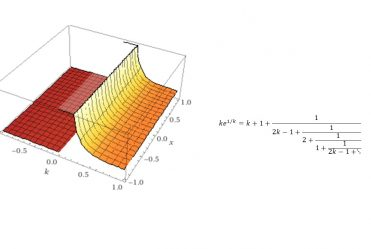Asst. Prof. Dr. Janyarak Tongsomporn​

My research interests are in the field of number theory and classical analysis especially in the stability of functional equations.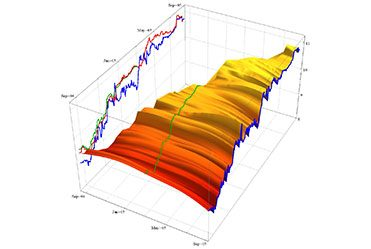Assoc. Prof. Dr. Sanae Rujivan

My research interests are in the area of mathematical finance, stochastic modeling and parameter estimation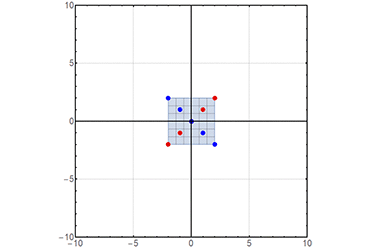Asst.Prof.Dr. Prathomjit Khachorncharoenkul

My research interests are in the area of linear algebra and its application. I study the structure of linear preservers on some spaces and the structure of matrices with some properties.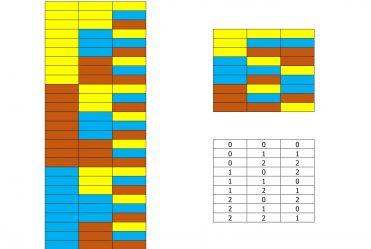Asst.Prof.Dr. Kittipong Laipaporn​

My research interests lie in the CLT by using Chen-Stein’s method and Fourier method, respectively. In my work, I use Stein’s method to derive rates on the uniform and non-uniform bound of convergence results which is focused on combinatorial designs and Fourier method to find the convergence conditions of infinitely-divisible distributions.Asst. Prof. Dr. Rasimate Maungchang

My research interests are in the area of geometric topology. I study some properties of surfaces such as length of curves, pants decompositions of surfaces. I am also interested in using visualizations to improve students’ learning in mathematics.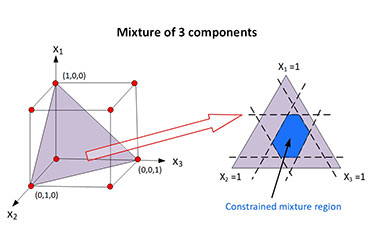Asst. Prof. Dr. Wanida Limmun

Statistical design of experiments is used extensively in a wide range of scientific and industrial investigations. I am interested in robust experimental designs and the related construction and regression analysis. Currently, I work mostly on the design of experiments in the situation where the independent factors are proportions of different components of a blend and the response is assumed to depend only on the relative proportions of the components in the mixture, the so-called mixture experiments.Asst. Prof. Dr. Phichet Jitjankarn

My research interests are in the area of transformation semigroups and coding theory.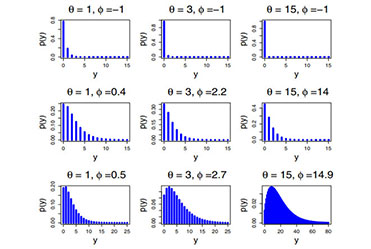Dr. Suttida Sangpoom

I create new probability distribution to present some of its mathematical properties. These properties are used to describe real data sets, for example, biological experiment data, stock price change data, education data, etc.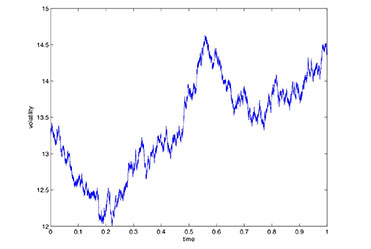Asst.Prof.Dr. Nopporn Thamrongrat

My research interests are in the area of high frequency data. I study volatility estimation and also study how to estimate the asymptotic (conditional) covariance matrix, which appears in many central limit theorems for stable laws in high-frequency estimation.

Asst.Prof. Dr. Kiattisak Prathom

My research is focused on both theoretical and applied mathematics. We use number theory and complex analysis to investigate the stability of mathematical modeling related to the cell cycle of budding yeast. Analyzing all zeros of convex combinations of polynomials is a key to understand the stability of mathematical models. Another research interest is finding closed-form formulas of recurrence differential equations that can be applied in financial and engineering fields.